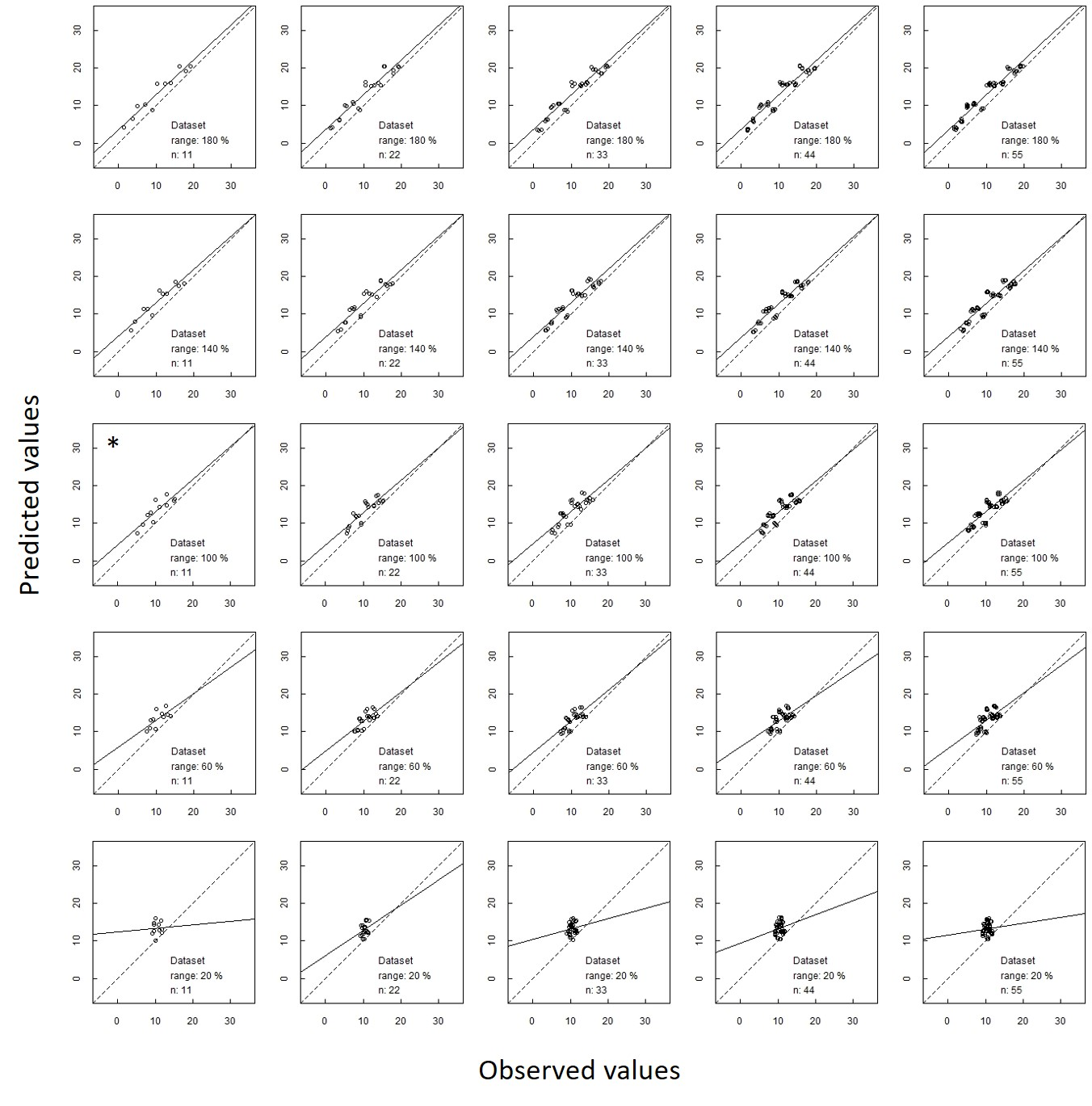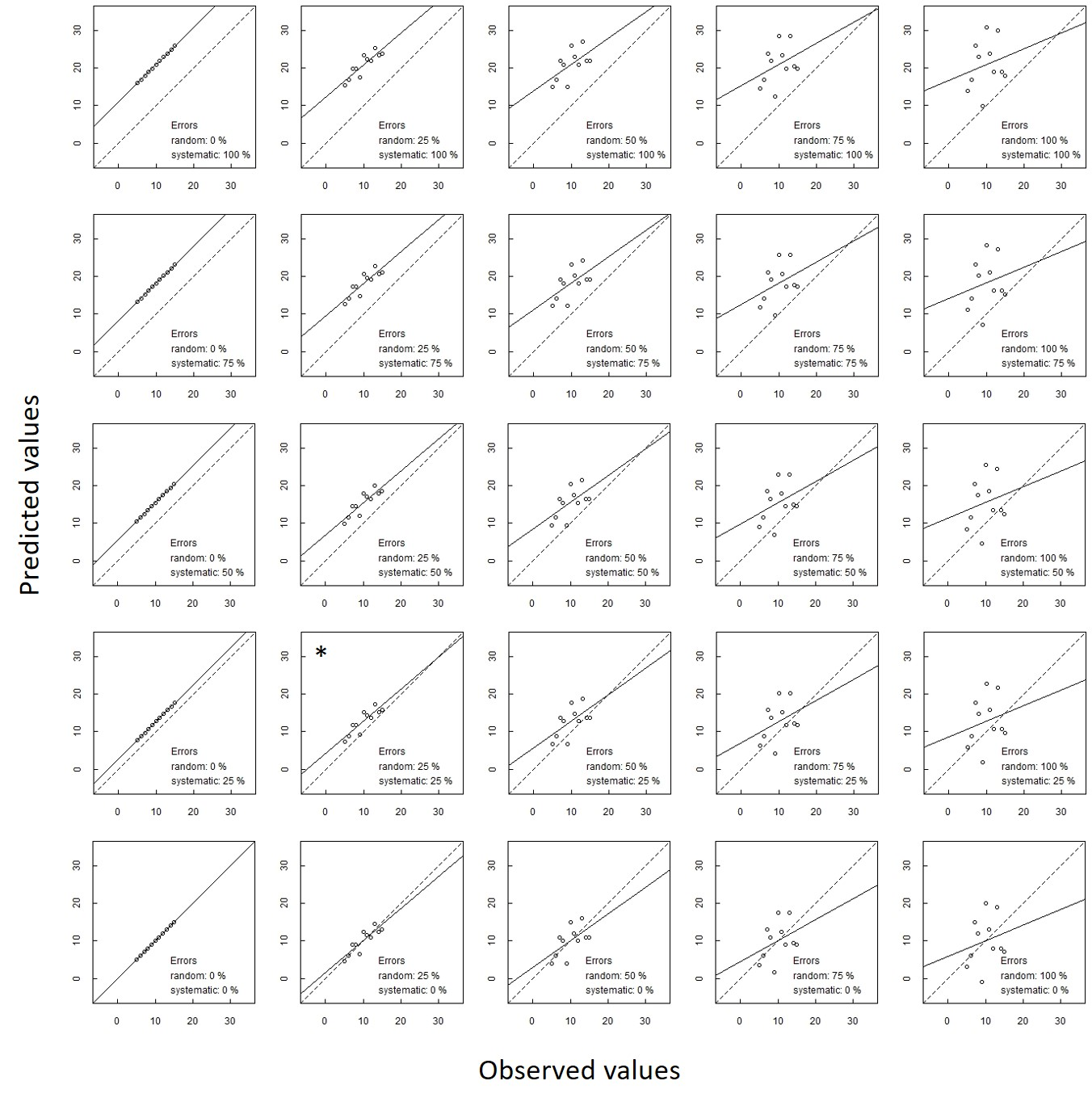## 1 Background

When models or maps are evaluated, validation metrics of model or map performance are commonly computed, based on vectors of observed values and their corresponding predicted values. In a systematic review of validation practices in the scientific literature within the subject area of digital soil mapping, about thirty different validation metrics were found to be used Piikki et al., 2021. These measures are sensitive to different aspects of model performance. Some are sensitive to random errors, others are sensitive to systematic error and yet others are sensitive to both (the total error). In addition, some metrics are sensitive to the range of the observed data and some are sensitive to the number of observations in the dataset. In addition, a few are constructed to be sensitive to the number of model parameters used in order to punish for model complexity. What the validation metrics are sensitive to, determine whether it is suitable to compare them between datasets or response variables (the modelled or mapped entity).

Functions to compute the validation metrics listed by Piikki et al. (in press) are provided as functions in the R package valmetrics. The present document is a simulation study aiming to demonstrate the sensitivities of the validation metrics to: i) different types of error (random errors, systematic errors and their combinations), and ii) different dataset properties (spread in observed values, number of observations (n) and their combinations).

This demonstration is based on synthetic datasets of predicted and observed values with different levels of random and systematic errors and with different ranges in observed values and different numbers of observations.

## 2 Materials and methods

### 2.1 A synthetic dataset for simulation of sensitivities to different types of error

First, a dataset of synthetic observed values was defined as all integers from 5 to 15. Then twenty-five different prediction sets were constructed, one for each of the orthogonal combinations of five levels (weights) of systematic errors and five levels of random errors. The synthetic predictions were computed according to:

$p_{ijk} = o_{ijk} + w_{ri} × e_{rk} + w_{sj} × e_{sk}$

where p is a vector of predicted values, o is a vector of observed value, w_r is a vector of weights of the random error, w_s is a vector of weights of the systematic error, e_r is a vector of random errors and e_s is a vector of systemic errors (a bias). The vectors o, e_r and e_s, all of length 11, are:

$o = [5, 6, 7, …, 15]$

$w_r = w_s = [0, 0.25, 0.5, 0.75, 1]$

$e_r = [0 , -2, 4, 2, -8, 6, -4, -6, 10, -10, 8]$

$e_s≈[11,11,11,…,11]$

The systematic error weights were: 0, 25 %, 50 %, 75 % and 100 %. The vector e_r was obtained by sampling an ordered vector of integers between -5 and 5 without replacement and multiplying by 2. The constant bias of 11 was chosen such that the systematic errors would be of the same magnitude as the random errors. The systematic error was a constant offset 2 times the mean of the absolute random errors:

$2 × mean(abs(e_r )) = 10.90909$

### 2.2 A synthetic dataset for simulation of sensitivities to dataset properties

The synthetic dataset for simulation of sensitivities to dataset properties was constructed for one selected level of systematic and random errors (w_r=w_s=0.25). The vector of observed data (o) was linearly scaled to the ranges: [1, 19], [3,17 ], [5, 15], [7,13] and [9, 11], i.e to ranges that are 20%, 60%, 100%, 140 % and 180% of the original range in observed values.

$ranges = [0.2, 0.6, 1, 1.4, 1.8]$

Then, predicted values was computed according to equation 1. The resulting dataset was multiplied 1, 2, 3, 4, or 5 times to get different numbers of observations n:

$n = [11, 22, 33, 44, 55]$

This means that 25 datasets, one for each combination of the five ranges and the five numbers of observations were constructed.

## 3 Results

### 3.1 The synthetic datasets

Plots of predicted values versus observed values in the synthetic datasets are presented in figures 1 and 2.{width=85%}{width=85%}

### 3.2 Simulation results

Figures 3 and 4 show 28 validation metrics for the 25 datasets in Figure 1 and the 25 datasets in Figure 2. In Figure 3, it is evident that ac, adjr2, aic , e, lc, lccc, mad , mae, mape, mare, msdr, mse, nmse, nrmse, nu , precision, rmdse, rmse, rpd, rpiq, smape and sse are sentitive to both random and systematic errors, while mde, mdse and me (also called bias) area sensitive only to systematic error and nu, r, r2 and sde are sensitive only to random error. The metrics are dented by their function names in the valmetrics R package. Equations are given by Piikki et al. (in press).